# Calculating the DSIM Data Set Size

The Delta Save Images (DSIM) data set must be large enough to hold an image of every Associator and Data Storage block written or changed by the database nucleus while an online save operation is active.

The DSIM data set can be any device type. If an Associator or Data Storage block to be stored in the DSIM data set does not fit into one DSIM block, it is split into two or more components. Using a device type with a block size large enough to prevent a split reduces the number of DSIM I/Os and thereby improves performance.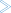The following sequence of calculations can be used to estimate and define the size, in cylinders, of the DSIM data set (DSIMSIZE):

1. First, determine the following factors:

 PLBLKS estimated number of PLOG blocks written during an online save operation. PLBLOKSIZ PLOG block size, by device type. This is available from the block tables in the Adabas Installation documentation . DSIMBSIZ DSIM data set block size for the DSIM device type. The DSIM device type is the same as the sort/temp device type. DSIMBCYL DSIM data set blocks per cylinder for the DSIM device type. Obtain the number by multiplying the "RABNs/track" table value by the "tracks/cylinder"; see the block tables in the Adabas Installation documentation. SECFAC security factor, a multiplier to ensure that no DSIM overrun occurs.
2. Convert the PLOG block count (PLBLKS) into a DSIM block count (DSIMBLKS):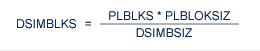When the PLOG block size and the DSIM block size are equal, then this calculation is not necessary; just set DSIMBLKS equal to PLBLKS.

3. Calculate a secured (using the security factor SECFAC) "raw" DSIM size, in blocks:

```
DSIMRAW = DSIMBLKS * SECFAC

```
4. Round the DSIM size, in blocks, to the next larger cylinder boundary: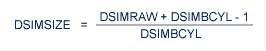The value DSIMSIZE is the calculated size of the DSIM data set, in cylinders.

#### Example:

If the average number of PLOG blocks written per save is 5000, based on a 3390 device type for both the PLOG and the DSIM data set, the DSIM size is calculated as follows: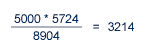The total "raw" DSIM block count is determined by multiplying the DSIM block count by the security factor. In this case, 2:

```
3214 * 2 = 6248

```

The "raw" block count is rounded up to the next 3390 cylinder boundary: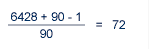or 72 cylinders for the DSIM data set.